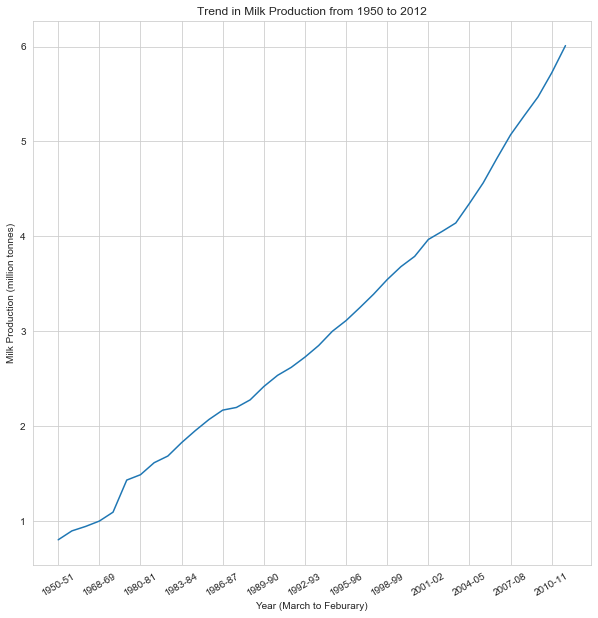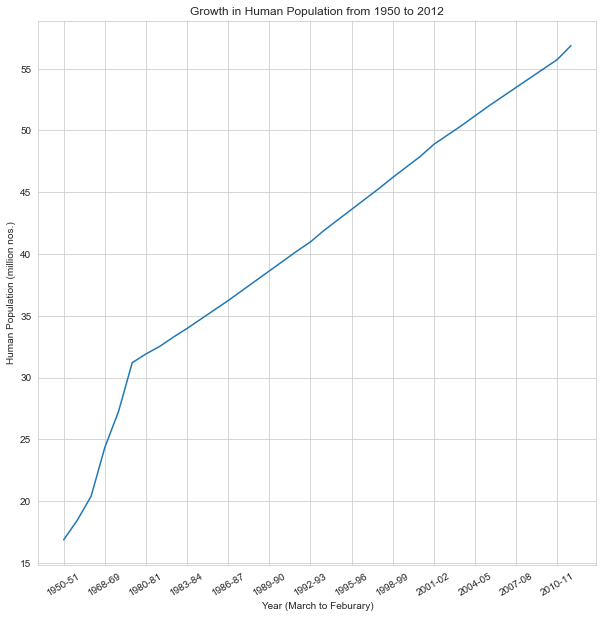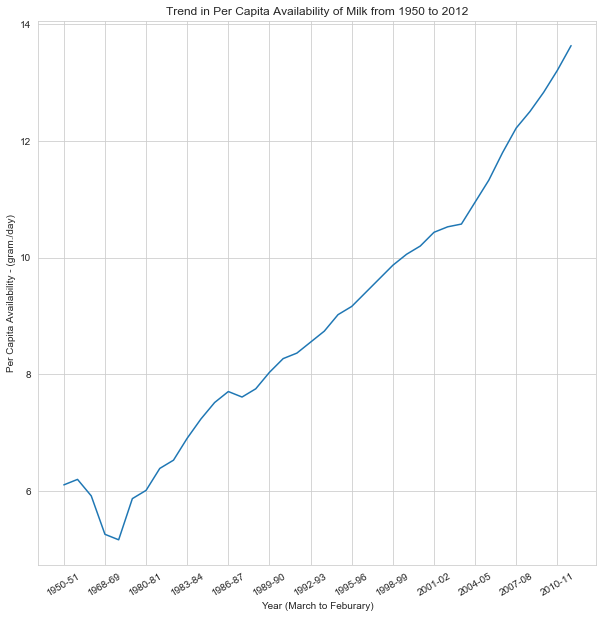This notebook was created as part of a case study done for the Internet of Things course attended in 5th Semester.
The amount of data received from Karnataka Co-operative Milk Federation was very limited.
The objective of this case study was to search for a way to centralize and automate the milk industry in India.

In :
``````import numpy as np
import pandas as pd

import matplotlib.pyplot as plt
import seaborn as sns

import jovian

%matplotlib inline
``````
In :
``````milk_df = pd.read_csv('MilkProduction_1951_2012_n.csv')
milk_df.columns
``````
Out:
``````Index(['Year (March to Feburary)', 'Milk Production (million tonnes)',
'Human Population (million nos.)',
'Per Capita Availability - (gram./day)'],
dtype='object')``````
In :
``milk_df.describe()``
Out:
In :
``````def trendplot(x,y,title):
plt.figure(figsize=(10,10))
plt.xticks(np.arange(0,60,3), rotation = 30)
plt.title(title)
sns.set_style("whitegrid")
sns.lineplot(x = x, y = y)
``````
In :
``````trendplot(x = milk_df['Year (March to Feburary)'],
y = milk_df['Milk Production (million tonnes)'],
title = 'Trend in Milk Production from 1950 to 2012'
)
``````In :
``````trendplot(x = milk_df['Year (March to Feburary)'],
y = milk_df['Human Population (million nos.)'],
title = 'Growth in Human Population from 1950 to 2012'
)
``````In :
``````trendplot(x = milk_df['Year (March to Feburary)'],
y = milk_df['Per Capita Availability - (gram./day)'],
title = 'Trend in Per Capita Availability of Milk from 1950 to 2012'
)
``````###### Trend in Milk Production from 1950 to 2012

We can infer the following from this graph:

• Milk production in the state of Karnataka has been linearly increasing throughout
• There is a small boost in milk production in 1970s
• Rate of Milk production has increased slightly since 2002-2003

###### Growth in Human Population from 1950 to 2012

We can infer the following from this graph:

• There has been a linear growth in population in Karnataka from 1950 to 1970
• Population growth has significantly slowed down since 1970 although there is still linear growth

###### Trend in Per Capita Availability of Milk from 1950 to 2012

We can infer the following from this graph:

• Availability of Milk in the state suffered a substantial drop during 1970s compared to 1950s
• It took upto a decade to recover similar availability of milk
• Availability of milk has been growing linearly since then and has slightly increased since 2002 - 2003
• This coincides with the beginning of White Revolution in the country stariing from the year 1970
In [ ]:
``jovian.commit()``
```[jovian] Saving notebook.. ```